Previous in Forum: Compressor Turbine Next in Forum: What's the Difference Between Trapezoidal Tooth and Circular Tooth Timing Belt?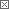#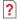Question About Drying Theory

12/03/2012 1:07 PM

I am studying drying theory by myself, using this book:

http://www.nzifst.org.nz/unitoperations/drying1.htm

and I have a question. In the problem that I copy below, I am not sure if the total heat supplied to the product is independent of the way it is supplied. I mean, is it the same to:

a) Evaporate all water at 24 ºC and then heating the steam in air to 71 ºC?

b) Heating all water up to 71 ºC and then evaporating?

c) Or some intermediate path?

I mean, is the total heat supplied dependent only on the starting and final states or does the path matter?

EXAMPLE 7.4. Efficiency of a potato dryer
A dryer reduces the moisture content of 100 kg of a potato product from 80% to 10% moisture. 250 kg of steam at 70 kPa gauge is used to heat 49,800 m3 of air to 80°C, and the air is cooled to 71°C in passing through the dryer. Calculate the efficiency of the dryer. The specific heat of potato is 3.43 kJ kg-1 °C-1. Assume potato enters at 24°C, which is also the ambient air temperature, and leaves at the same temperature as the exit air.

In 100 kg of raw material there is 80% moisture, that is 80 kg water and 20 kg dry material,
total weight of dry product = 20 x (10/9)
= 22.2 kg
weight of water = (22.2 - 20)
= 2.2 kg.
water removed = (80 - 2.2)
= 77.8 kg.

Heat supplied to potato product
= sensible heat to raise potato product temperature from 24°C to 71°C + latent heat of vaporization.

Now, the latent heat of vaporization corresponding to a saturation temperature of 71°C is 2331 kJ kg-1
Heat (minimum) supplied/100 kg potato
= 100 x (71 - 24) x 3.43 + 77.8 x 2331
= 16 x 103 + 181 x 103
= 1.97 x 105 kJ.
Heat to evaporate water only = 77.8 x 2331
= 1.81 x 105 kJ

The specific heat of air is 1.0 J kg-1 °C-1 and the density of air is 1.06 kg m-3 (Appendix 3)
Heat given up by air/100 kg potato
= 1.0 x (80 - 71) x 49,800 x 1.06
= 4.75 x 105 kJ.

The latent heat of steam at 70 kPa gauge is 2283 kJ kg-1
Heat in steam = 250 x 2283
= 5.71 x 105 kJ.

Therefore (a) efficiency based on latent heat of vaporisation only:
= (1.81 x 105)/ (5.71 x 105)
= 32%
(b) efficiency assuming sensible heat remaining in food after drying is unavailable
= (1.97 x 105)/ (5.71 x 105)
= 36%
(c) efficiency based heat input and output, in drying air
= (80 - 71)/ (80 - 24)
= 16%

Whichever of these is chosen depends on the objective for considering efficiency. For example in a spray dryer, the efficiency calculated on the air temperatures shows clearly and emphatically the advantages gained by operating at the highest feasible air inlet temperature and the lowest air outlet temperatures that can be employed in the dryer.

__________________
I am happy.
Interested in this topic? By joining CR4 you can "subscribe" to

Check out these comments that don't yet have enough votes to be "official" good answers and, if you agree with them, rate them!
Guru

Join Date: Feb 2011
Posts: 7025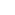#1

###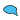Re: Question About Drying Theory

12/03/2012 2:43 PM

..............another homework question....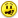#4

###Re: Question About Drying Theory

12/05/2012 5:33 AM

Not really. But I know it sounds like one.

__________________
I am happy.
Off Topic (Score 5)
Commentator

Join Date: Nov 2012
Location: Sunnyvale, CA
Posts: 62#2

###Re: Question About Drying Theory

12/03/2012 5:31 PM

Barring losses to different leakage paths, then yes, the total heat supplied depends only on the starting and final states, independent of path. How could it be otherwise? It would violate conservation of energy.

That said, leakage paths are often significant, and there may be practical reasons to prefer one path over another. One also has to be careful in defining the initial and final states. In your paths a) and b), for example, you only mention water, steam and air. However, in the potato drying problem you're trying to work, there's also potato material to consider. Path a) leaves the potato at 24 C throughout the process, and only heats the water vapor at the end. Path b) heats the water -- and the potato with it -- to 71 C, leaving it there. So the initial and final states are not, in fact, identical.

As a side note, the definition of "efficiency" in this type of calculation is problematic. From a rigorous thermodynamic perspective, the efficiency for any drying process will be close to zero; no work is produced and almost no change in potential energy. There is a small change in potential energy from the heat of hydration of the potato mass, but it's tiny compared to the energy used to dehydrate the potato in any realistic system. Nor is its value specified, or easily determined from theory.

Since the rigorous thermodynamic definition is useless for practical engineering, a looser utilitarian definition is used. The minimum energy for an idealized but quasi-realistic process is determined. In your potato drying example, it would be the latent energy of vaporization, at 24 C, of 2.2 kg of water per 100 kg of potato. That energy is then compared to the actual energy consumed in the process under evaluation to derive what might be termed the "functional efficiency" for the process. But note that there's an implicit assumption that the heat of vaporization is always unrecoverable.

That's not necessarily a valid assumption. One can imagine a vacuum drying system in which water vapor is pumped from the drying chamber, boosted to a slightly higher pressure, and condensed on a surface that transfers heat back into the drying chamber. The transferred heat balances the cooling from the evaporation of water in the drying chamber. This is a heat pumping process that could theoretically yield a functional efficiency of several hundred percent, compared to the non-condensing reference model.

Hope that helps. It's a good thing I'm retired, because that was a \$50 answer if I were still billing my hours.#5

###Re: Question About Drying Theory

12/05/2012 5:40 AM

Thank you very much. That is an amazing answer.

__________________
I am happy.
Guru

Join Date: Oct 2008
Posts: 42328#3

###Re: Question About Drying Theory

12/03/2012 9:59 PM

If this is a small family operation air drying would be most cost efficient.

But, drying time is important in any commercial operation. If throughput is to be maximized, then heat is dictated. So, cost of production in total is most important.

I don't have an opinion about the formulas.

Interested in this topic? By joining CR4 you can "subscribe" to